Matter Matter is ANYTHING that has mass and

• Slides: 16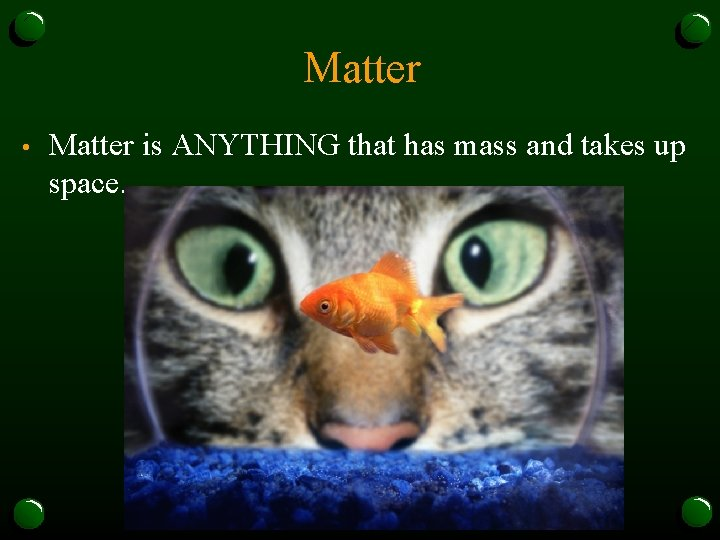Matter • Matter is ANYTHING that has mass and takes up space.Matter Is All Around Us • Where is it in our classroom? • • • Lab Tables Chairs People Pencils Books Even the air.All Matter Has Mass • How do we find out how much matter and object contains? • • • Measure the mass. What tool do we use? What unit? Grams (g)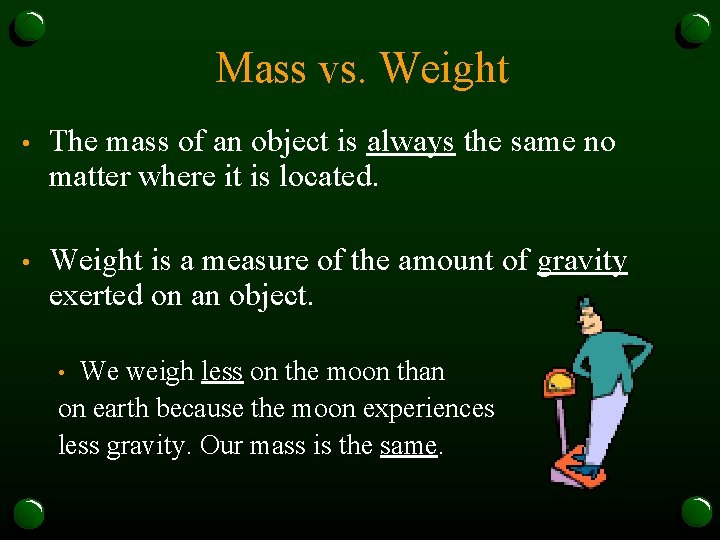Mass vs. Weight • The mass of an object is always the same no matter where it is located. • Weight is a measure of the amount of gravity exerted on an object. We weigh less on the moon than on earth because the moon experiences less gravity. Our mass is the same. •All Matter Has Volume • • The amount of space the matter occupies What tools do we use? What Units? • Liters (L) OR • Cubic centimeters (cm 3 ) Remember 1 m. L = 1 cm 3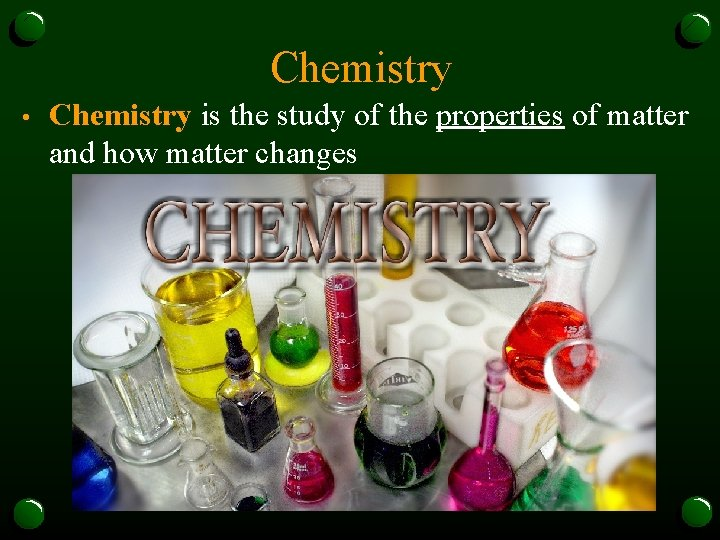Chemistry • Chemistry is the study of the properties of matter and how matter changesPhysical Properties • A Physical Property is a characteristic of a substance that can be observed without changing it into another substance. • • Color, Shape, Oder, Texture, Volume, Mass, Density, Conductivity, State A Physical Change is a change that affects one or more physical properties of a substance. • • If I rip a piece of paper up it’s still paper just a different shape If I dye water green it’s still water just water with green dye in it.How do I know if it’s a physical change? • When deciding whether a change is a physical change or not, ask yourself whether you have the same substance you started with. • If the substance is the same, then the changes it underwent were all physical changes.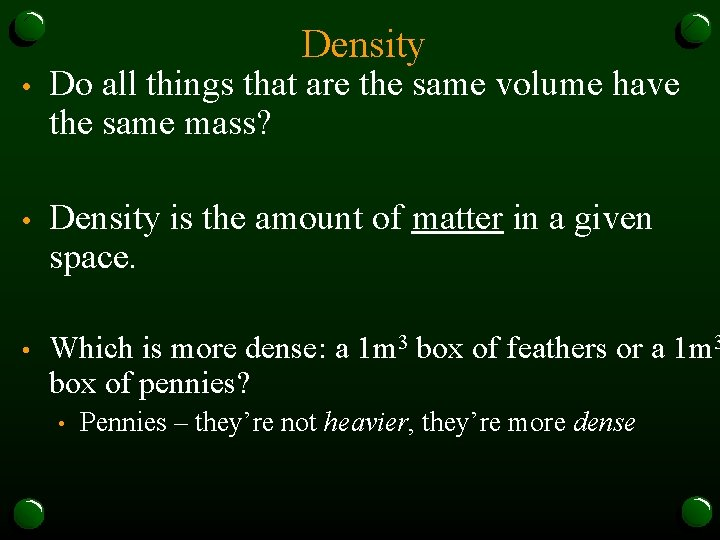Density • Do all things that are the same volume have the same mass? • Density is the amount of matter in a given space. • Which is more dense: a 1 m 3 box of feathers or a 1 m 3 box of pennies? • Pennies – they’re not heavier, they’re more denseCalculating Density • • Density = Mass Volume M D X V The unit for density is g/cm 3 or g/ml• Density is useful for identifying substances for two reasons: Density stays the same for any SIZE piece of something • The density of one substance is usually different from that of another substance. •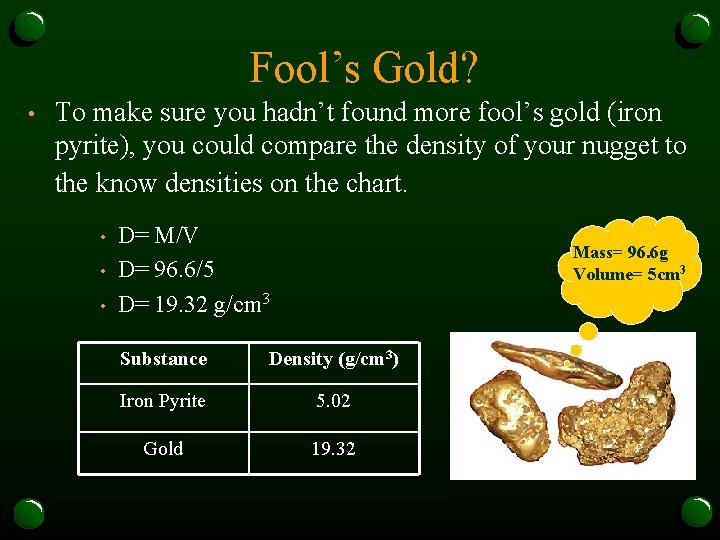Fool’s Gold? • To make sure you hadn’t found more fool’s gold (iron pyrite), you could compare the density of your nugget to the know densities on the chart. • • • D= M/V D= 96. 6/5 D= 19. 32 g/cm 3 Mass= 96. 6 g Volume= 5 cm 3 Substance Density (g/cm 3) Iron Pyrite 5. 02 Gold 19. 32Brian. Pop ReviewTry it out! A glass marble has a volume of 5 cm 3 and a mass of 13 g. What is the density of glass? What do you know? Volume = 5 cm 3, mass = 13 g Write the formula: D = m/v Substitute into the formula: D = 13 g 5 cm 3 Calculate: D = 2. 6 g/cm 3 Check that your units agree: Unit is g/cm 3. Unit of density is g/cm 3. Units agree. Answer: D = 2. 6 g/cm 3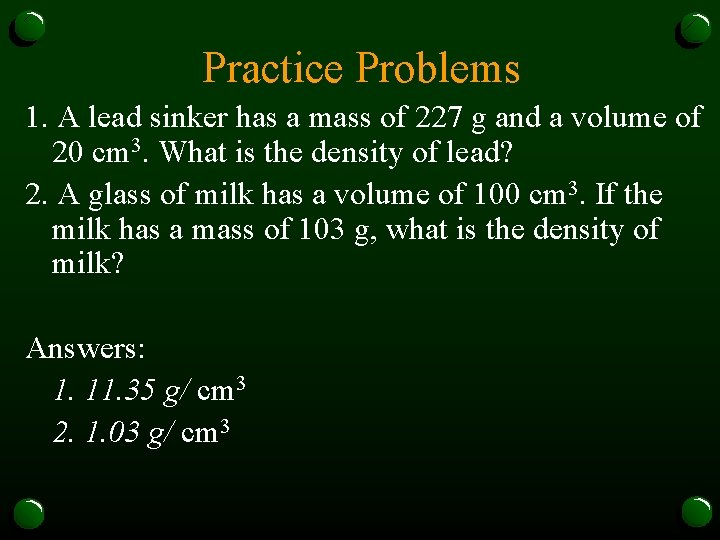Practice Problems 1. A lead sinker has a mass of 227 g and a volume of 20 cm 3. What is the density of lead? 2. A glass of milk has a volume of 100 cm 3. If the milk has a mass of 103 g, what is the density of milk? Answers: 1. 11. 35 g/ cm 3 2. 1. 03 g/ cm 3